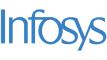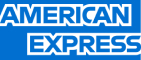New update is available. Click here to update.
Topics

# Anagram Pairs

Moderate0/80
Average time to solve is 30m+65 more companies

## Problem statement

You are given two strings 'str1' and 'str1'.

You have to tell whether these strings form an anagram pair or not.

The strings form an anagram pair if the letters of one string can be rearranged to form another string.

Pre-requisites:

``````Anagrams are defined as words or names that can be formed by rearranging the letters of another word. Such as "spar" can be formed by rearranging letters of "rasp". Hence, "spar" and "rasp" are anagrams.
``````

Other examples include:

``````'triangle' and 'integral'
'listen' and 'silent'
``````
Note:
``````Since it is a binary problem, there is no partial marking. Marks will only be awarded if you get all the test cases correct.
``````
Detailed explanation ( Input/output format, Notes, Images )
Sample Input 1:
``````rasp spar
``````
Sample Output 1:
``````True
``````
Explanation For Sample Output 1:
``````Since both the strings have one 'a', one 'p', one 'r', and one 's', they are anagrams.
``````
Sample Input 2:
``````rasp spaz
``````
Sample Output 2:
``````False
``````
Constraints:
``````1 <= N <= 10^5
1 <= M <= 10^5
Where N and M are the lengths of the strings str1 and str2 , respectively.

Time Limit: 1sec
``````
``````Can you solve this in O( N ) time?